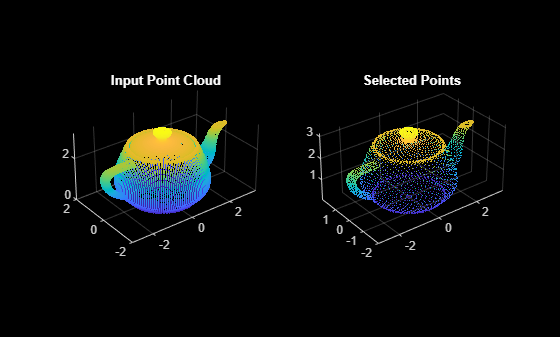# select

Select points in point cloud

## Syntax

``ptCloudOut = select(ptCloud,indices) ``
``ptCloudOut = select(ptCloud,row,column)``
``ptCloudOut = select(___,'OutputSize',outputSize)``

## Description

example

````ptCloudOut = select(ptCloud,indices) `returns a `pointCloud` object containing only the points that are selected using linear indices.```
````ptCloudOut = select(ptCloud,row,column)` returns a `pointCloud` object containing only the points that are selected using row and column subscripts. This syntax applies only if the input is an organized point cloud data of size M-by-N-by-3.```
````ptCloudOut = select(___,'OutputSize',outputSize)` returns the selected points as a `pointCloud` object of size specified by `outputSize`.```

## Examples

collapse all

Read a point cloud data into the workspace.

`ptCloud = pcread('teapot.ply');`

Read the number of points in the point cloud data.

`Length = ptCloud.Count;`

Select indices within the range [1, `Length`].

```stepSize = 10; indices = 1:stepSize:Length;```

Select points specified by the indices from input point cloud. This selection of points downsamples the input point cloud by a factor of `stepSize`.

`ptCloudB = select(ptCloud,indices);`

Display the input and the selected point cloud data.

```figure subplot(1,2,1) pcshow(ptCloud) title('Input Point Cloud','Color',[1 1 1]); subplot(1,2,2) pcshow(ptCloudB) title('Selected Points','Color',[1 1 1]);```## Input Arguments

collapse all

Point cloud, specified as a `pointCloud` object.

Indices of selected points, specified as a vector.

Row indices, specified as a vector. This argument applies only if the input is an organized point cloud data of size M-by-N-by-3.

Column indices, specified as a vector. This argument applies only if the input is an organized point cloud data of size M-by-N-by-3.

Size of the output point cloud, `ptCloudOut`, specified as `'selected'` or `'full'`.

• If the size is `'selected'`, then the output contains only the selected points from the input point cloud, `ptCloud`.

• If the size is `'full'`, then the output is same size as the input point cloud `ptCloud`. Cleared points are filled with `NaN` and the color is set to [0 0 0].

## Output Arguments

collapse all

Point cloud, returned as a `pointCloud` object.